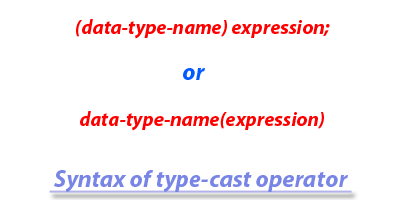< Prev
Next >

# C++ Type Cast Operator

C++ provides us a type-cast operator which allows us to explicit cast the value of an arithmetic expression to get the precise result by avoiding the data loss. Before we understand what exactly a type-cast operator is about, we need to understand some important arithmetic expressions rules in C++ language-

• The result of an arithmetic operation between two or more primitive integral(non-decimal) data-type always results in an integral data-type value.

• The result of an arithmetic operation between two or more primitive floating data-type always results in a floating data-type value.

• The result of an arithmetic operation between a primitive integral data-type and a floating primitive data-type always results in a floating data-type value.

To understand the rules we have just explained, let us see an example.
``````#include<iostream>

using namespace std;

int main()
{
int a = 10, b =3;

//An arithmetic expression involving two int variables a and b
float result = a/b;

cout << "The result of dividing 10/3 is : " << div;
}``````

## Output

``The value of dividing 10/3 is : 3``

According to the one of the rules we have just explained- "The result of an arithmetic operation between two or more primitive integral(non-decimal) data-type always results in integral data-type value", hence in the program above, the result of a division operation involving two int variables a(10) and b(3) has resulted in an int value i.e. 3, even though we were expecting the correct result to be a decimal value i.e. a float value, 3.3333.

To avoid the data loss in such scenarios, we need to use the type-cast operator to explicit cast the value of an expression from one data-type to another data-type.

## Using type-cast operator

The type-cast operator is used to explicitly cast the value of an expression from one data-type to another data-type. In order to use the type-cast operator, let us see its syntax -``````//C++ Example of type-cast operator

#include<iostream>

using namespace std;

int main()
{
int a = 10, b =3;

//Using the type-case operator to cast the result of an arithmetic expression
//involving two int variables, to float
float result = (float)a/b;

cout << "The result of dividing 10/3 is : " << result;
}``````

## Output

``The result of dividing 10/3 is : 3.33333``

In the program above, we have used the type-cast operator to cast the result of arithmetic division operation between two int values a(10) and b(3) to get its precise decimal value result i.e. float value, 3.3333, without losing the fractional part.

< Prev
Next >
< Scope Resolution Operator
New Operator in C++ >